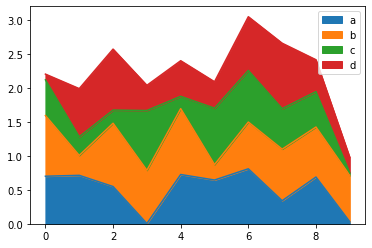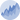# Pandas Stacked Area Chart

This post explains how to create a stacked area plot using the `pandas` library of python.

Using pandas library, the stacked area charts are plotted with the `plot.area()` function. Each column of your data frame will be plotted as an area on the chart.

``````# libraries
import pandas as pd
import numpy as np
import matplotlib.pyplot as plt

# Dataset
df = pd.DataFrame(np.random.rand(10, 4), columns=['a', 'b', 'c', 'd'])

# plot
df.plot.area()

# show the graph
plt.show()``````## Contact & Edit

👋 This document is a work by Yan Holtz. Any feedback is highly encouraged. You can fill an issue on Github, drop me a message onTwitter, or send an email pasting `yan.holtz.data` with `gmail.com`.